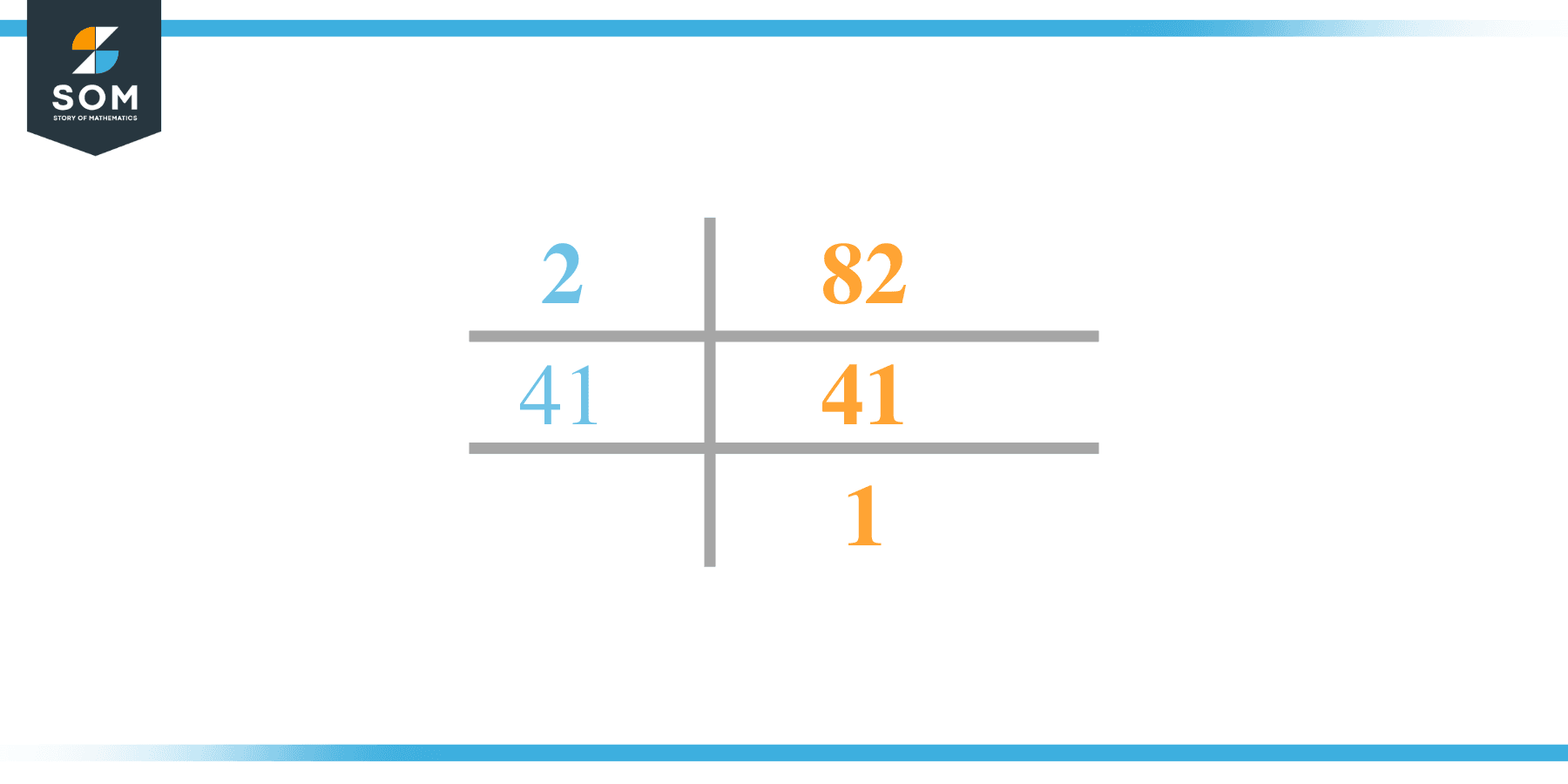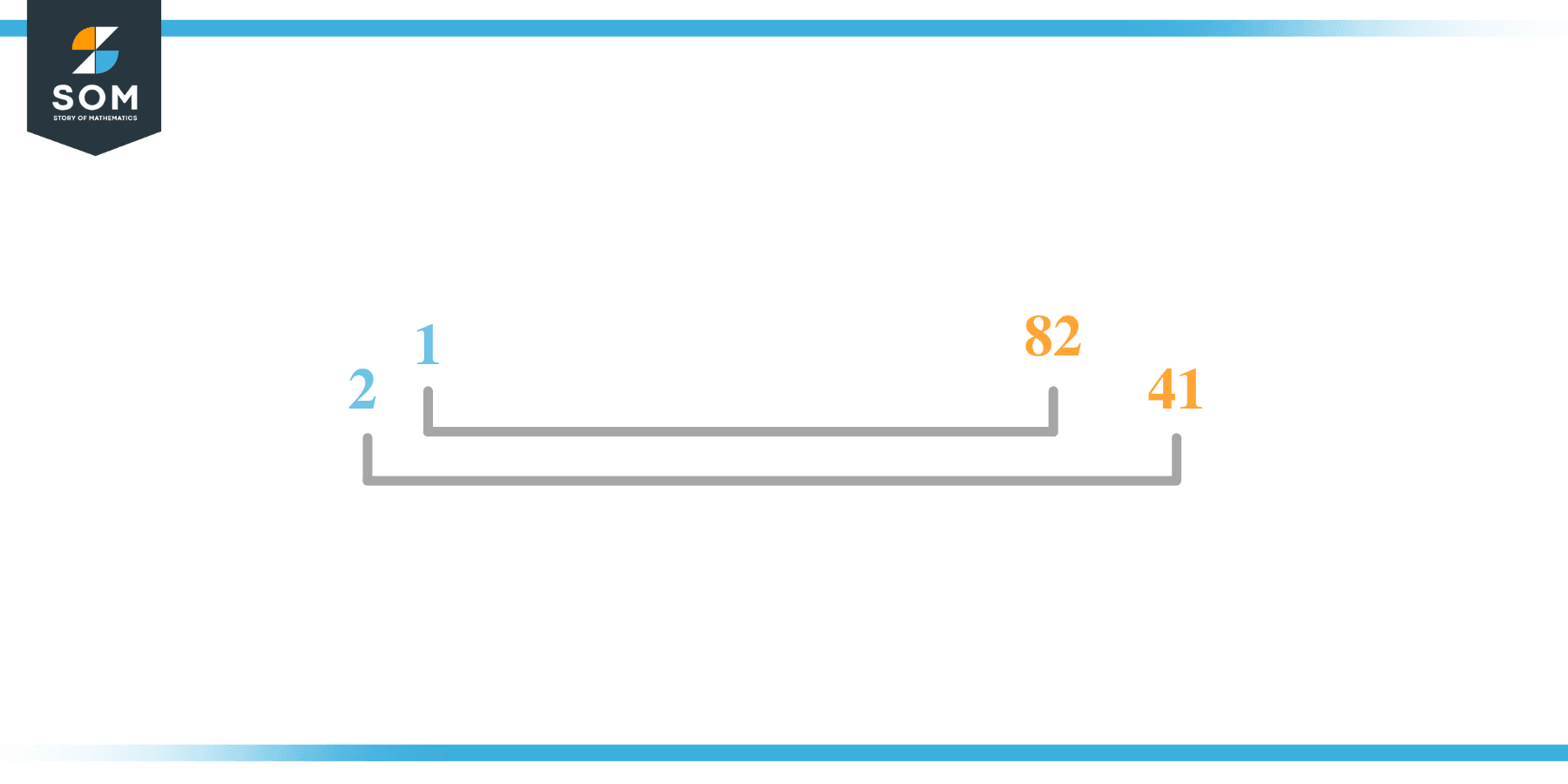# Factors of 82: Prime Factorization, Methods, and Examples

The factors of 82 are the group of natural numbers that completely divide 82 with a zero remainder. 82 is an even number. Another interesting fact about number 82 is that it is a composite number, meaning it has more than two factors.The factors of 82 can be positive and negative provided that the product of any of those two is always the factored number.

### Factors of 82

Here are the factors of number 82.

Factors of 82: 1, 2, 41, and 82

### Negative Factors of 82

The negative factors of 82 are similar to its positive factors, just with a negative sign.

Negative Factors of 82: -1, -2, -41, and -82

### Prime Factorization of 82

The prime factorization of 82 is the way of expressing a number’s prime factors in the form of its product.

Prime Factorization of 82: 2 x 41

In this article, we will learn about the factors of 82 and how to find them using various techniques such as upside-down division, prime factorization, and factor tree.

## What Are the Factors of 82?

The factors of number 82 are 1, 2, 41, and 82. Both of these numbers are the factors as they do not leave any remainder when divided by 82.

The prime factors of 82 are 2 and 41. The prime factors of the number 82 can be determined using the technique of prime factorization.

## How To Find the Factors of 82?

You can find the factors of 82 by using the rules of divisibility. The rule of divisibility states that any number when divided by any other natural number then it is said to be divisible by the number if the quotient is the whole number and the resulting remainder is zero.

To find the factors of 82, create a list containing the numbers that are exactly divisible by 82 with zero remainders. One important thing to note is that 1 and numbers themselves are always the factors of that particular factored number as every natural number has 1 and the number itself as its factor.

1 is also called the universal factor of every number. The factors of 67 are determined as follows:

$\dfrac{82}{1} = 82$

$\dfrac{82}{2} = 41$

$\dfrac{82}{41} = 2$

$\dfrac{82}{82} = 1$

Therefore, 1, 2, 41, and 82 are the factors of 82.

### Total Number of Factors of 82

For 82 there are 4 positive factors as found above and 4 negative factors. So in total, there are 8 factors of 82.

To find the total number of factors of the given number, follow the procedure mentioned below:

1. Find the factorization of the given number.
2. Demonstrate the prime factorization of the number in the form of exponent form.
3. Add 1 to each of the exponents of the prime factor.
4. Now, multiply the resulting exponents together. This obtained product is equivalent to the total number of factors of the given number.

By following this procedure the total number of factors of 71 is given as:

Factorization of 82 is 1 x 2 x 41.

The exponent of 1, 2, and 41 is 1.

Adding 1 to each and multiplying them together results in 8.

Therefore, the total number of factors of 82 is 8.

### Important Notes

Here are some important points that must be considered while finding the factors of any given number:

• The factor of any given number must be a whole number.
• The factors of the number cannot be in the form of decimals or fractions.
• Factors can be positive as well as negative.
• Negative factors are the additive inverse of the positive factors of a given number.
• The factor of a number cannot be greater than that number.
• Every even number has 2 as its prime factor which is the smallest prime factor.

## Factors of 82 by Prime FactorizationThe number 82 is a prime number. Prime factorization is a useful technique for finding the number’s prime factors and expressing the number as the product of its prime factors.

Before finding the factors of 82 using prime factorization, let us find out what prime factors are. Prime factors are the factors of any given number that are only divisible by 1 and themselves.

To start the prime factorization of 82, start dividing by its smallest prime factor. First, determine that the given number is either even or odd. If it is an even number, then 2 will be the smallest prime factor. Since 82 is an even number, therefore, start dividing 82 by 2.

Continue splitting the quotient obtained until 1 is received as the quotient. The prime factorization of 82 can be expressed as:

82 = 1 x 2 x 41

## Factors of 82 in PairsThe factor pairs are the duplet of numbers that when multiplied together result in the factorized number. Depending upon the total number of factors of the given numbers, factor pairs can be more than one.

82 is a composite number with four factors therefore it has 2 positive factor pairs.

For 82, the factor pairs can be found as:

1 x 82 = 82

2 x 41 = 82

The possible factor pairs of 82 are (1, 82) and (2, 41).

Both of these numbers in pairs, when multiplied, give 82 as the product.

The negative factor pairs of 82 are given as:

-1 x -82 = 82

-2 x -41 = 82

It is important to note that in negative factor pairs, the minus sign has been multiplied by the minus sign due to which the resulting product is the original positive number. Therefore, -1, -2, -41, and -82 are called negative factors of 82.

The list of all the factors of number 82 including positive as well as negative numbers is given below

Factor List of 82: 1, -1, 2, -2, 41, -41, 82, and -82

## Factors of 82 Solved Examples

To better understand the concept of factors, let’s solve some examples.

### Example 1

How many factors of 82 are there?

### Solution

The total number of Factors of 82 is 8. Positive factors are 1, 2, 41, and 82.

Negative factors are -1, -2, -41, and -82.

### Example 2

Find the factors of 82 using prime factorization.

### Solution

The prime factorization of 82 is given as:

82 $\div$ 2 = 41

41 $\div$ 41 = 1

So the prime factorization of 71 can be written as:

2 x 41 = 82

### Example 3

What is the sum of factors of number 82?

### Solution

The sum of the factors of 82 is 1 + 2 + 41 + 82 = 126.

Therefore the sum is 126.